# Introduction

Everyone who works with Telecom knows there is a relationship between Watts and dBm. But if the Power is expressed in Watts, why we must know - and use - this relationship in our day-to-day?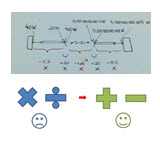Let’s try to understand today in a simple manner, and discover why the use of decibels help us much.

# Watt (W) and miliWatt (mW)

First of all, to understand what it means for example 0 dBm, we at least have to know the basic unit of power, the Watt. By definition, 1 Watt means 1 Ampere (A) current in 1 Volt (V) voltage, or in mathematical terms P = VA. It is interesting to note that the amount of power radiated by an antenna is very small in terms of Watts, but it is enough to reach several miles.And as the signs are very small, is more common to refer to them in terms of prefix, such as military or milliwatts (mW), which means 1 / 1000 (one thousandth) of Watts.

# Mathematics

Besides the signals were rather small, it - as well as other quantities of physics such as electricity, heat or sound - propagate nonlinearly. It would be more or less like compound interest on a loan.

Or brought into our world of engineers, imagine a cable for transmitting 100 watts, with a loss of 10% per meter. If the spread was linear, the final 10 meters would have no more power!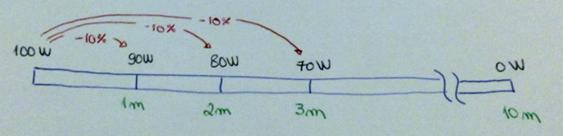Only it’s not how it happens. In the first meters, have 10% less power, which is 90 watts. And this is the value that ‘enter’ on the cable until the next meter. Thus, the second meter, we would have 10% less of that power or 81 watts (= 90 - 9). Repeating this calculations, you see that in fact the power never reaches zero, as it would if calculations were linear. (At the end of the cable have actually 34.86 Watt)To solve problems o deal with this - and make our lives easier - we need to know the logarithms. We saw this in school, but there are people who do not like to hear. The good thing is that we need not know much about them, just understand what they are.

Just understand that if we transformed the magnitudes in logarithms, the calculations become addition and subtraction rather than multiplication and division.Of course, in order to do the calculations by adding and subtracting, we must make the necessary conversions. But with the help of a calculator or Excel, is not that complicated.

# Decibels (dB)

By definition, we have:Sure, we say that working with logarithms (or decibels) is much easier - and the common good. But by the formulas above, still can not understand. So the best way to understand why we use dB (decibels), is seeing how they help us through a practical example.

Consider a standard wireless link, where we have a transmitter (1) and a receiver (5), Antennas (3), Cables, Jumpers and Connectors (2) and Free Space (4).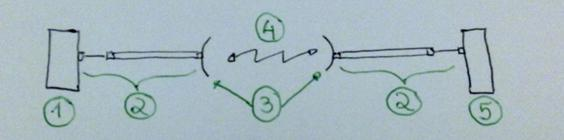Using real values, and without using the help of dB, let’s do the math and see, from the transmitted power, how much power we have at receiver. So with dummy values, but close to reality, we have:

• Transmitter Power = 40 Watts
• Cables and connectors loss = - 0.5 (Half Power)
• Antenna Gain = 20 + times in the Power
• Free Space Loss = - 0 000 000 000 000 000 1 Power

Note: This amount of loss in free space is quite big. And it is obtained based on distance and other factors. For now, just accept that it is a practical value of loss of RF for a given distance of our link.

The link with the absolute values in Watt would then be as below.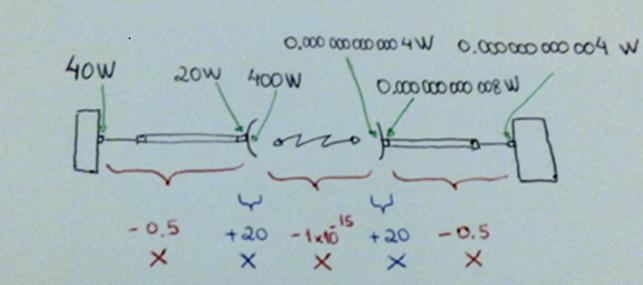We can work this way, of course. But you must agree that it is not very friendly.

Now, if we use the proper conversion of power, gain and loss for dB, we can simply add and subtract.It was so much easier, isn’t it?

Now we just need to know the formulas to do the conversions.

# Converting with Formulas in Excel

Considering that the amount of wattage is in cell B3, the formula for convrting W in dBm is:

= 10 * ( LOG10 ( 1000 * B3 ) )

And the formula to reverse - convert dBm to Watt, considering that our power in dBm is in cell B6 is:

= 10 * ( LOG10 ( 1000 * B6 ) )

And as a result, we have calculated values.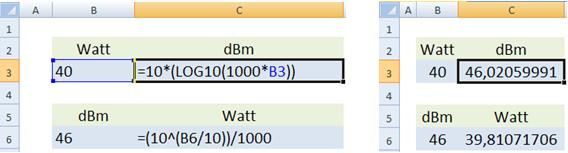Note that in case we are using the 1000 value in the formulas, for wearing the Watt, but we want the result in dBm.

To calculate (convert) db to ratio, or ratio to db, the formulas do not include the value of 1000.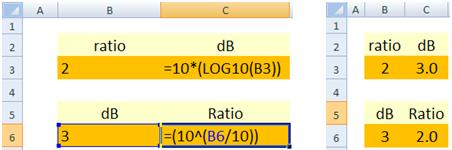# Calculations without using a calculator

Of course, we will use calculators in the projects and programs such as Excel. But we also know how to do calculations (conversions) without using a calculator. If anyone tells you that the power is + 46 dBm, you need to know what that means in terms of Watts.

For this, there are certain tricks that can be used to arrive at least an approximate match.

For this, a good way is to memorize the equivalent to multiplying factors in dB, as in the table below (at least those that are in bold).With the corresponding values of dB and multiplier factor, we convert eg +46 dBm to mW.

Answer: First, we expressed 46 values that we already know by heart.

So 46 = 10 + 10 + 10 + 10 + 3 + 3

That is, we multiply the reference value (1 mW) for four times the factor of 10 and twice the factor of 2.

What gives us

1mW x 10 x 10 x 10 x 10 = 1 000 0 mW

1 000 0 mW x 2 x 2 = 40000 mW = 40 W

Ie, + 46 dBm is equal to 40 watts.

# Conclusion

Well, I think now you have given to see that when we do the calculations in dB everything is easier. Moreover, the vast majority of Telecom equipment has specifications of its units in dB (Power, Gain, Loss, etc.).

In short, just use basic math to understand the values and reach the final figures.

When we say that such a signal is attenuated by 3 dB, means that the final power is half the initial power. Likewise, if a given power is increased by 3 dB means twice the power.

A good practice, irrespective of how you will work with the calculations is to store at least some values such as 0 dBm = 1 mWatt (our initial question), 30 dBm = 1 Watt, and in our example, 46 dBm = 40 Watt.

So you can quickly learn, for example, the equivalent for the calculations.

For example, 43 dBm = 46 dBm - 3 dB. That is, half the power of 46 dbm. Then, 43 dBm = 20 Watts!

Just finally, in our example, the received power was - 84 dBm, remember?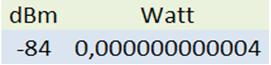In this case, doesn’t need memorizing. Just so you know which is equivalent to a very low power, but enough for a good example for GSM call.